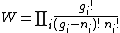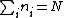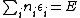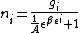# Fermi-Dirac statistics

Also found in: Dictionary, Thesaurus, Wikipedia.

## Fermi-Dirac statistics,

class of statistics that applies to particles called fermions. Fermions have half-integral values of the quantum mechanical property called spin and are "antisocial" in the sense that two fermions cannot exist in the same state. Protons, neutrons, electrons, and many other elementary particles are fermions. See Bose-Einstein statisticsBose-Einstein statistics,
class of statistics that applies to elementary particles called bosons, which include the photon, pion, and the W and Z particles. Bosons have integral values of the quantum mechanical property called spin and are "gregarious" in the sense that an
; elementary particleselementary particles,
the most basic physical constituents of the universe. Basic Constituents of Matter

Molecules are built up from the atom, which is the basic unit of any chemical element. The atom in turn is made from the proton, neutron, and electron.
; statistical mechanicsstatistical mechanics,
quantitative study of systems consisting of a large number of interacting elements, such as the atoms or molecules of a solid, liquid, or gas, or the individual quanta of light (see photon) making up electromagnetic radiation.
.

## Fermi-Dirac statistics

The statistical description of particles or systems of particles that satisfy the Pauli exclusion principle. This description was first given by E. Fermi, who applied the Pauli exclusion principle to the translational energy levels of a system of electrons. It was later shown by P. A. M. Dirac that this form of statistics is also obtained when the total wave function of the system is antisymmetrical. See Exclusion principle

Such a system is described by a set of occupation numbers ni which specify the number of particles in energy levels εi. It is important to keep in mind that εi represents a finite range of energies, which in general contains a number, say gi, of nondegenerate quantum states. In the Fermi statistics, at most one particle is allowed in a nondegenerate state. (If spin is taken into account, two particles may be contained in such a state.) This is simply a restatement of the Pauli exclusion principle, and means that nigi. The probability of having a set &lcub;ni&rcub; distributed over the levels εi, which contain gi nondegenerate levels, is described by Eq. (1), which gives just the number

(1)of ways that ni can be picked out of gi, which is intuitively what one expects for such a probability. The equilibrium state which actually exists is the set of n's that makes W a maximum, under the auxiliary conditions given in Eqs. (2a) and (2b).
(2{\em a})(2{\em b})These conditions express the fact that the total energy E and the total number of particles N are given. Equation (3)
(3)holds for this most probable distribution. Here A and β are parameters, to be determined from Eq. (3); in fact, β = 1/kT, where k is Boltzmann's constant and T is the absolute temperature. When the 1 in the denominator may be neglected, Eq. (3) goes over into the Boltzmann distribution.

Classical conditions pertain when the volume per particle is much larger than the volume associated with the de Broglie wavelength λ of a particle. For electrons in a metal at 300 K, the ratio of the volume per particle to λ3 has the value 10-4, showing that classical statistics fail altogether. When the classical distribution fails, a degenerate Fermi distribution results. A somewhat lengthy calculation yields the result that in this case the contribution of the electrons to the specific heat is negligible. This resolves an old paradox, for, according to the classical equipartition law, the electronic specific heat C should be (3/2)Nk, whereas in reality it is very small. See Bose-Einstein statistics, Kinetic theory of matter, Quantum statistics, Statistical mechanics

McGraw-Hill Concise Encyclopedia of Physics. © 2002 by The McGraw-Hill Companies, Inc.
The following article is from The Great Soviet Encyclopedia (1979). It might be outdated or ideologically biased.

## Fermi-Dirac Statistics

the quantum statistics applicable to systems of identical particles with half-integral spin, that is with spin 1/2, 3/2,... in units of Planck’s constant ℏ. Fermi-Dirac statistics was proposed by E. Fermi in 1926, and its quantum-mechanical meaning was elucidated by P. Dirac in the same year.

In quantum mechanics the state of a system is described by the system’s wave function, which depends on the coordinates and spins of all its particles. For a system of fermions, that is, particles obeying Fermi-Dirac statistics, the wave function is antisymmetric. In other words, it changes its sign when any two identical particles are interchanged. W. Pauli proved in 1940 that the type of statistics applicable to a system of particles is determined by the particle spin: an assembly of particles with half-integral spin obeys Fermi-Dirac statistics, and an assembly of particles with integral spin obeys Bose-Einstein statistics. According to Fermi-Dirac statistics, no more than one fermion can occupy each quantum state (the Pauli exclusion principle).

For an ideal gas of fermions, or Fermi gas, in equilibrium, the average number i of particles in a state of energy Ei is determined by the Fermi-Dirac distribution function: i = 1/(e(Ei – μ)/kT + 1), where i denotes the set of quantum numbers that characterize the state of the particle, k is the Boltzmann constant, T is the absolute temperature of the gas, and μ is the chemical potential. Fermi-Dirac statistics is applicable to Fermi gases and Fermi liquids.

D. N. ZUBAREV

## Fermi-Dirac statistics

[¦fer·mē di¦rak stə′tis·tiks]
(statistical mechanics)
The statistics of an assembly of identical half-integer spin particles; such particles have wave functions antisymmetrical with respect to particle interchange and satisfy the Pauli exclusion principle.
McGraw-Hill Dictionary of Scientific & Technical Terms, 6E, Copyright © 2003 by The McGraw-Hill Companies, Inc.
References in periodicals archive ?
Let us then introduce Fermi-Dirac statistics for electrons.
Thus we have the Fermi-Dirac statistics that the statistical electron propagator is of the form i/[[gamma][p.sup.[mu]]-m which is the propagator of the Dirac equation and is the electron propagator of the conventional QED.
Let us then introduce the Fermi-Dirac statistics on the electron and we consider the on-mass-shell case as in the conventional QED.
As a Fermi-Dirac statistics we have shown in the above Section that the vertex term -ie([p.sub.E] + [q.sub.E]) is replaced with the vertex term -ie([p.sub.E] + [q.sub.E]) [[gamma].sup.[mu]]/2.
Let us then consider the Fermi-Dirac statistics on the one-loop vertex correction (118).
By Fermi-Dirac statistics the factor ([p.sub.E] + [q.sub.E]) in (129) of [([p.sub.E] + [q.sub.E]).sup.2] gives the statistical term [([p.sub.E] + [q.sub.E])1/2 [[gamma].sup.[mu]].
If we denote fermion with (f) and boson with (b), and because g =1 for generalized Fermi-Dirac statistics and g =0 for generalised Bose-Einstein statistics, then it follows that the wholeness of both (f) and (b).

Site: Follow: Share:
Open / Close